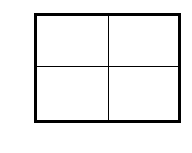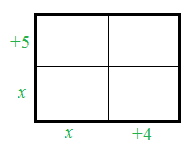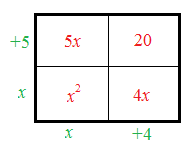### Home > AC > Chapter 5 > Lesson 5.2.1 > Problem5-70

5-70.

Find each of the following products by drawing and labeling a generic rectangle or by using the Distributive Property.

1.  $( x + 5 ) ( x + 4 )$

Create a generic rectangle. A generic rectangle: A rectangle with 4 quadrants. Values within the rectangle indicate area. Values on the outside edge represent length and width of the rectangle.

Put the products on the outside of the rectangle.  A generic rectangle where the outside upper left edge is + 5 and the lower left edge is X. The bottom left edge is X and the bottom right edge is + 4.

Find the area of the smaller rectangles. The same rectangle in hint 2 with the interior labeled as follows: Upper left box is 5 X. Upper right box is 20. Lower left box is X squared. Lower right box is 4 X.

Write the areas as a sum.
$x^2+5x+4x+20$.

$(x+5)(x+4)=x^2+9x+20$1.  $2y(y+3)$

Follow the steps in part (a).